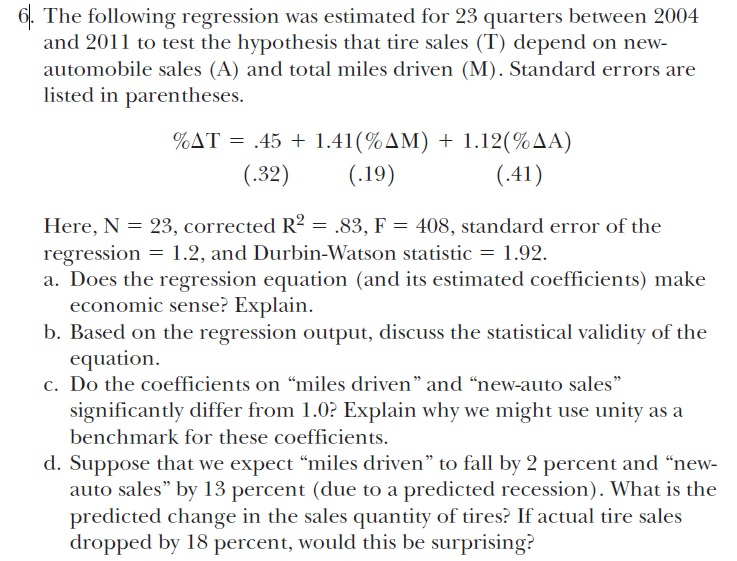Chat Now

# This is all one question Please show as much work as possible and explain where you can Image for The following regression was estimated for 23 quarters between 2004 and 2011 to test the hypothesis

### This is all one question Please show as much work as possible and explain where you can Image for The following regression was estimated for 23 quarters between 2004 and 2011 to test the hypothesis

This is all one question. Please show as much work as possible and explain where you can.Show transcribed image text The following regression was estimated for 23 quarters between 2004 and 2011 to test the hypothesis that tire sales (T) depend on new- automobile sales (A) and total miles driven (M). Standard errors are listed in parentheses. Here, N = 23, corrected R^2 = .83, F = 408, standard error of the regression = 1.2, and Durbin-Watson statistic = 1.92. a. Does the regression equation (and its estimated coefficients) make economic sense? Explain. b. Based on the regression output, discuss the statistical validity of the equation. c. Do the coefficients on ''miles driven'' and ''new-auto sales'' significantly differ from 1.0? Explain why we might use unity as a benchmark for these coefficients. d. Suppose that we expect ''miles driven'' to fall by 2 percent and ''new- auto sales'' by 13 percent (due to a predicted recession). What is the predicted change in the sales quantity of tires? If actual tire sales dropped by 18 percent, would this be surprising?

abhinav behal 11-May-2020Get solution